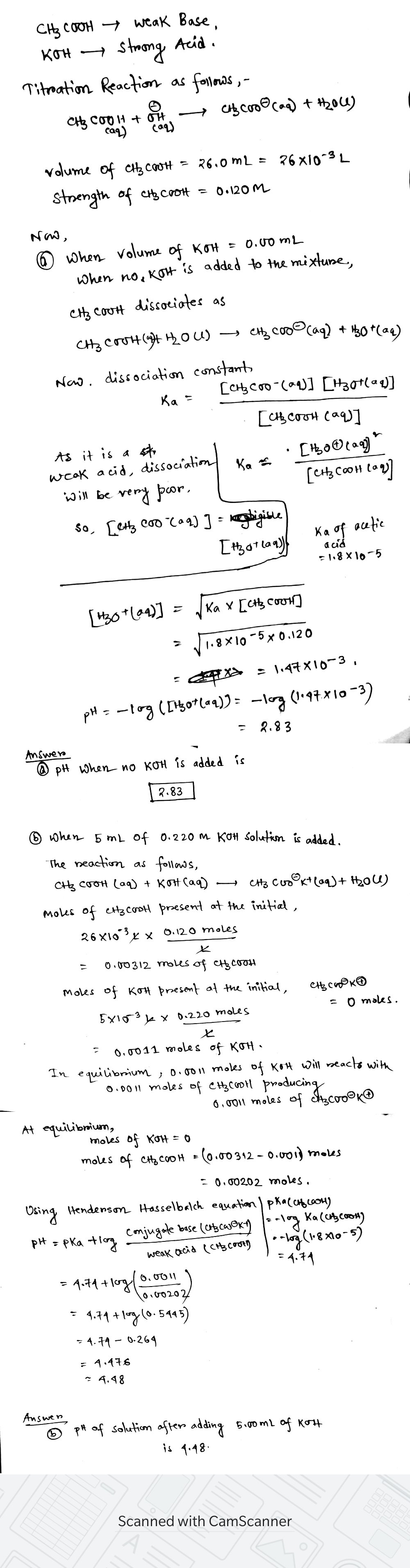In: Chemistry

# A 26.0−mL solution of 0.120 M CH3COOH is titrated with a 0.220 M KOH solution. Calculate...

A 26.0−mL solution of 0.120 M CH3COOH is titrated with a 0.220 M KOH solution. Calculate the pH after the following additions of the KOH solution:

(a) 0.00 mL

(b) 5.00 mL

## Solutions

##### Expert Solution## Related Solutions

##### A 25.0−mL solution of 0.100 M CH3COOH is titrated with a 0.200 M KOH solution. Calculate...
A 25.0−mL solution of 0.100 M CH3COOH is titrated with a 0.200 M KOH solution. Calculate the pH after the following additions of the KOH solution (a) 10.0 mL (b) 12.5 mL (c) 15.0 mL
##### A 25.0 mL solution of 0.100 M CH3COOH is titrated with a 0.200 M KOH solution....
A 25.0 mL solution of 0.100 M CH3COOH is titrated with a 0.200 M KOH solution. Calculate the pH after the following additions of the KOH solution: (a) 0.0 mL, (b) 5.0 mL, (c) 10.0 mL, (d) 12.5 mL, (e) 15.0 mL. (25 points)
##### A 25.0 mL solution of 0.100 M CH3COOH is titrated with a 0.200 M KOH solution....
A 25.0 mL solution of 0.100 M CH3COOH is titrated with a 0.200 M KOH solution. Calculate the pH after the following additions of the KOH solution: (a) 0.0 mL, (b) 5.0 mL, (c) 10.0 mL, (d) 12.5 mL, (e) 15.0 mL. (25 points)
##### A 50.0 mL solution of 0.167 M KOH is titrated with 0.334 M HCl . Calculate...
A 50.0 mL solution of 0.167 M KOH is titrated with 0.334 M HCl . Calculate the pH of the solution after the addition of each of the given amounts of HCl . 0.00 mL pH = 7.00 mL pH = 12.5 mL pH = 19.0 mL pH = 24.0 mL pH = 25.0 mL pH = 26.0 mL pH = 31.0 mL pH =
##### A 20.0-mL sample of 0.150 M KOH is titrated with 0.125 M HClO4 solution. Calculate the...
A 20.0-mL sample of 0.150 M KOH is titrated with 0.125 M HClO4 solution. Calculate the pH after the following volumes of acid have been added. 22.5 mL of the acid
##### A 83.0 mL sample of 0.0500 M HNO3 is titrated with 0.100 M KOH solution. Calculate...
A 83.0 mL sample of 0.0500 M HNO3 is titrated with 0.100 M KOH solution. Calculate the pH after the following volumes of base have been added. (a) 11.2 mL pH = _________ (b) 39.8 mL pH = __________    (c) 41.5 mL pH = ___________ (d) 41.9 mL pH = ____________    (e) 79.3 mL pH = _____________
##### 25.0 mL of a 0.100 M HCN solution is titrated with 0.100 M KOH. Ka of...
25.0 mL of a 0.100 M HCN solution is titrated with 0.100 M KOH. Ka of HCN=6.2 x 10^-10. a)What is the pH when 25mL of KOH is added (this is the equivalent point). b) what is the pH when 35 mL of KOH is added.
##### 1) A volume of 80.0 mL of a 0.560 M HNO3 solution is titrated with 0.230 M KOH. Calculate the volume of KOH required to reach the equivalence point.
1) A volume of 80.0 mL of a 0.560 M HNO3 solution is titrated with 0.230 M KOH. Calculate the volume of KOH required to reach the equivalence point. 2) 100. mL of 0.200 M HCl is titrated with 0.250 M NaOH. What is the pH of the solution after 50.0 mL of base has been added? AND What is the pH of the solution at the equivalence point? 3) Determine the pH at the equivalence point for the...
##### A 625 mL solution of HBr is titrated with 1.51 M KOH. If it takes 728.5...
A 625 mL solution of HBr is titrated with 1.51 M KOH. If it takes 728.5 mL of the base solution to reach the equivalence point, what is the pH when only 137 mL of the base has been added to the solution? I am getting an answer of .1018, but it's wrong
##### partb a)A 50.0-mL volume of 0.15 M HBr is titrated with 0.25 M KOH. Calculate the...
partb a)A 50.0-mL volume of 0.15 M HBr is titrated with 0.25 M KOH. Calculate the pH after the addition of 13.0 mL of KOH. Express your answer numerically. Part b) A 75.0-mL volume of 0.200 M NH3 (Kb=1.8×10−5) is titrated with 0.500 M HNO3. Calculate the pH after the addition of 15.0 mL of HNO3. Express your answer numerically.Part c) A 52.0-mL volume of 0.35 M CH3COOH (Ka=1.8×10−5) is titrated with 0.40 M NaOH. Calculate the pH after the...